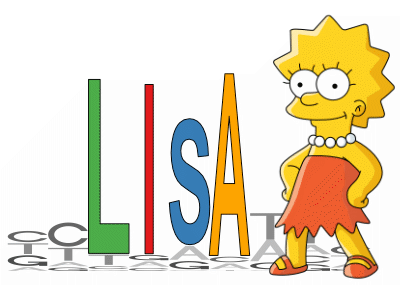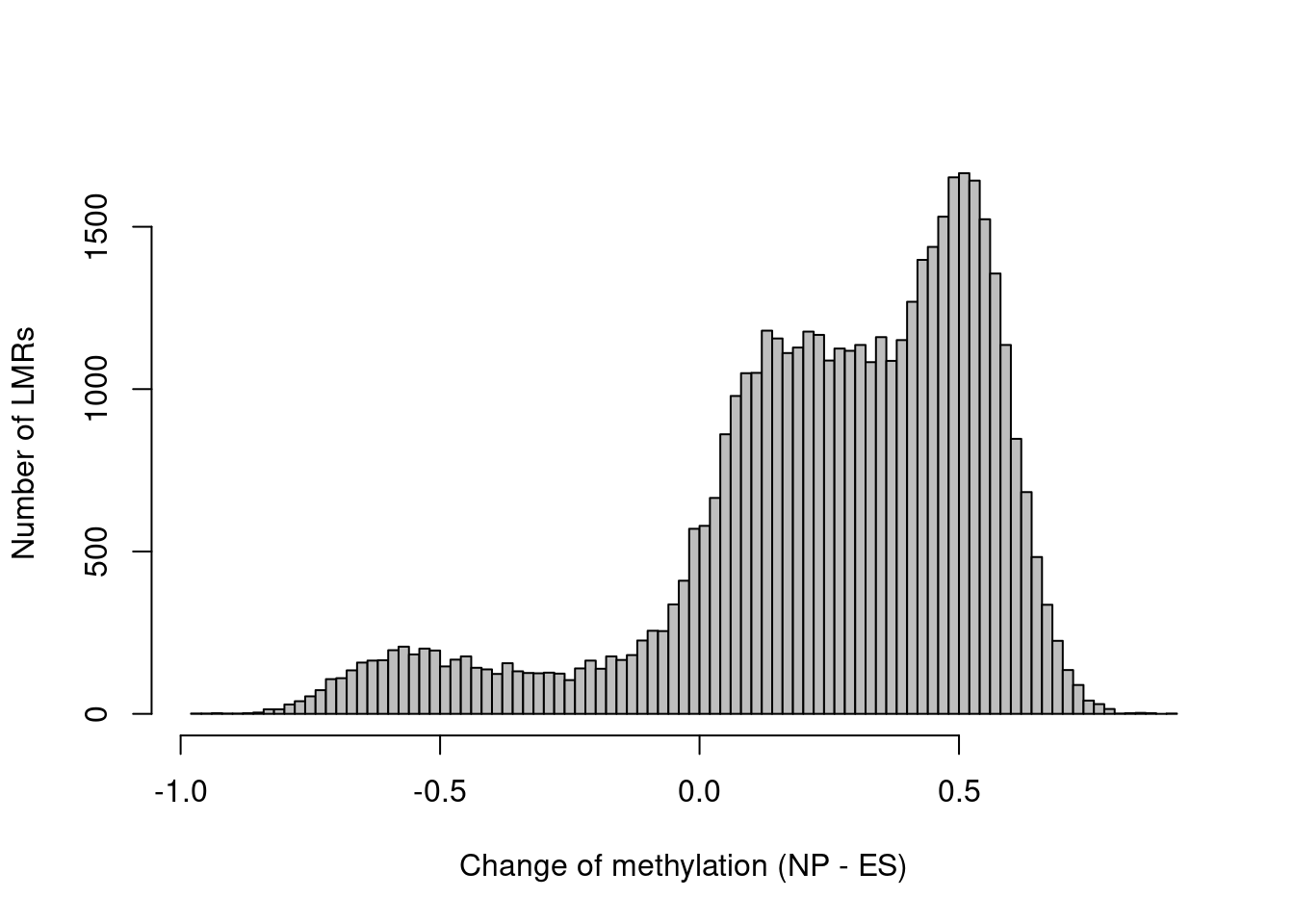1 Introduction

monaLisa is a collection of functions for working with biological sequences and motifs that represent the binding preferences of transcription factors or nucleic acid binding proteins.

For example, monaLisa can be used to conveniently find motif hits in sequences (see section 6), or to identify motifs that are likely associated with observed experimental data. Such analyses are supposed to provide potential answers to the question “Which transcription factors are the drivers of my observed changes in expression/methylation/accessibility?”.

Several other approaches have been described that also address this problem, among them REDUCE (Roven and Bussemaker 2003) and ISMARA (Balwierz et al. 2014). In monaLisa, we aim to provide a flexible implementation that integrates well with other Bioconductor resources, makes use of the sequence composition correction developed for Homer (Heinz et al. 2010) or stability selection (Meinshausen and Bühlmann 2010) and provides several alternative ways to study the relationship between experimental measurements and sequence motifs.

You can use known motifs from collections of transcription factor binding specificities such as JASPAR2020, also available from Bioconductor. Genomic regions could be for example promoters, enhancers or accessible regions for which experimental data is available.

Two independent approaches are implemented to identify interesting motifs:

• In binned motif enrichment analysis (monaLisa::calcBinnedMotifEnrR, see section 4), genomic regions are grouped into bins according to a numerical value assigned to each region, such as the change in expression, accessibility or methylation. Motif enrichments are then calculated for each bin, normalizing for differences in sequence composition in a very similar way as originally done by Homer (Heinz et al. 2010). The binned motif enrichment approach was first introduced in Ginno et al. (2018) and subsequently applied in e.g. Barisic et al. (2019). To see more details on how calcBinnedMotifEnrR resembles Homer, check the function help page. We recommend using this function to do the binned motif enrichment analysis, since it corrects for sequence composition differences similarly to Homer, but is implemented more efficiently. monaLisa::calcBinnedMotifEnrHomer implements the same analysis using Homer and therefore requires a local installation of Homer, and monaLisa::calcBinnedKmerEnr(see section 5) implements the analysis for k-mers instead of motifs, to study sequence enrichments without the requirement of known motifs.

• Randomized Lasso stability selection (monaLisa::randLassoStabSel, see the stability selection vignette, monaLisa uses a robust regression approach (stability selection, Meinshausen and Bühlmann (2010)) to predict what transcription factors can explain experimental measurements, for example changes in chromatin accessibility between two conditions. Also this approach allows to correct for sequence composition. In addition, similar motifs have to “compete” with each other to be selected.

For both approaches, functions that allow visualization of obtained results are provided.

If you prefer to jump right in, you can continue with section 3 that shows a quick hypothetical example of how to run a binned motif enrichment analysis. If you prefer to actually compute enrichments on real data, you can find below a detailed example for a binned motif enrichment analysis (section 4).

2 Installation

monaLisa can be installed from Bioconductor via the BiocManager package:

if (!requireNamespace("BiocManager", quietly = TRUE))
install.packages("BiocManager")

BiocManager::install("monaLisa")

3 Quick example: Identify enriched motifs in bins

The quick example below, which we do not run, illustrates how a binned motif enrichment analysis can be performed in monaLisa. We assume that you already have extracted a set of peaks. The sequences of the peak regions are stored in a Biostrings::DNAStringSet (peak_seqs), and additionally each peak is associated with a numeric value (e.g., the change of methylation between two conditions, stored in the peak_change vector), that will be used to bin the regions before finding motifs enriched in each bin.

library(monaLisa)

# bin regions
# (peak_change is a numerical vector)
# (peak_change needs to be created by the user to run this code)
peak_bins <- bin(x = peak_change, binmode = "equalN", nElement = 400)

# calculate motif enrichments
# (peak_seqs is a DNAStringSet, pwms is a PWMatrixList)
# (peak_seqs and pwms need to be created by the user to run this code)
se <- calcBinnedMotifEnrR(seqs = peak_seqs,
bins = peak_bins,
pwmL = pwms)

The returned se is a SummarizedExperiment with assays negLog10P, negLog10Padj, expForegroundWgt, pearsonResid, log2enr, sumForegroundWgtWithHits and sumBackgroundWgtWithHits, each containing a matrix with motifs (rows) by bins (columns). The values are:

• negLog10P: the raw P value ($$-\log_{10} p$$) of a given motif enrichment in a given bin. Each P value results from an enrichment calculation comparing occurrences of each motif in the bin to its occurrences in background sequences, defined by the background argument (by default: sequences in all other bins).
• negLog10Padj: Same as negLog10P but adjusted for multiple testing using the method of provided in the p.adjust.method argument, by default: Benjamini and Hochberg, 1995 (p.adjust(..., method="fdr")).
• expForegroundWgt: The expected number of regions in the bin containing a given motif.
• pearsonResid: Standardized Pearson residuals, a measure of motif enrichment akin to a z-score for the number of regions in the bin containing the motif. The standardized Pearson residuals are given by
$$resid = (o - \mu)/\sigma$$, where $$\mu$$ is the expected count and $$\sigma$$ the standard deviation of the expression in the numerator, under the null hypothesis that the probability of containing a motif is independent of whether the sequence is in the foreground or the background (see e.g.  Agresti (2007), section 2.4.5).
• log2enr: Motif enrichments, calculated as: $$log2enr = log2((o + c)/(e + c))$$, where $$o$$ and $$e$$ are the observed and expected numbers of regions in the bin containing a given motif, and $$c$$ is a pseudocount defined by the pseudocount.log2enr argument.
• sumForegroundWgtWithHits and sumBackgroundWgtWithHits are the sum of foreground and background sequences that have at least one occurrence of the motif, respectively. The background sequences are weighted in order to adjust for differences in sequence composition between foreground and background.

In addition, rowData(se) and colData(se) give information about the used motifs and bins, respectively. In metadata(se) you can find information about parameter values.

4 Binned motif enrichment analysis: Finding TFs enriched in differentially methylated regions

This example is based on an in vitro differentiation system, in which mouse embryonic stem (ES) cells are differentiated into neuronal progenitors (NP). In an earlier study (Stadler et al. 2011), we have analyzed the genome-wide CpG methylation patterns in these cell types and identified so called low methylated regions (LMRs), that have reduced methylation levels and correspond to regions bound by transcription factors.

We also developed a tool that systematically identifies such regions from genome-wide methylation data (Burger et al. 2013). Interestingly, a change in methylation of LMRs is indicative of altered transcription factor binding. We will therefore use these regions to identify transcription factor motifs that are enriched or depleted in LMRs that change their methylation between ES and NP cell states.

library(GenomicRanges)
library(SummarizedExperiment)
library(JASPAR2020)
library(TFBSTools)
library(BSgenome.Mmusculus.UCSC.mm10)
library(monaLisa)
library(ComplexHeatmap)
library(circlize)

4.2 Genomic regions of interest

monaLisa contains a file with genomic coordinates (mouse mm10 assembly) of LMRs, with the respective changes of methylation:

lmrfile <- system.file("extdata", "LMRsESNPmerged.gr.rds",
package = "monaLisa")
lmr
#> GRanges object with 45414 ranges and 1 metadata column:
#>           seqnames          ranges strand |    deltaMeth
#>              <Rle>       <IRanges>  <Rle> |    <numeric>
#>            chr1 3549153-3550201      * |    0.3190299
#>            chr1 3680914-3682164      * |    0.0657352
#>            chr1 3913315-3914523      * |    0.4803313
#>            chr1 3953500-3954157      * |    0.4504727
#>            chr1 4150457-4151567      * |    0.5014768
#>       ...      ...             ...    ... .          ...
#>        chrY 4196254-4196510      * | -0.020020382
#>        chrY 4193654-4194152      * | -0.102559935
#>        chrY 4190208-4192766      * | -0.031668206
#>        chrY 4188072-4188924      * |  0.130623049
#>        chrY 4181867-4182624      * |  0.000494588
#>   -------
#>   seqinfo: 21 sequences from an unspecified genome

We can see there are 45414 LMRs, most of which gain methylation between ES and NP stages:

hist(lmr$deltaMeth, 100, col = "gray", main = "", xlab = "Change of methylation (NP - ES)", ylab = "Number of LMRs")In order to keep the computation time reasonable, we’ll select 10,000 of the LMRs randomly: set.seed(1) lmrsel <- lmr[ sample(x = length(lmr), size = 10000, replace = FALSE) ] 4.3 Bin genomic regions Now let’s bin our LMRs by how much they change methylation, using the bin function. We are not interested in small changes of methylation, say less than 0.3, so we’ll use the minAbsX argument to create a no-change bin in [-0.3, 0.3). The remaining LMRs are put into bins of 800 each: bins <- bin(x = lmrsel$deltaMeth, binmode = "equalN", nElement = 800,
minAbsX = 0.3)
table(bins)
#> bins
#> [-0.935,-0.242]  (-0.242,0.327]   (0.327,0.388]   (0.388,0.443]   (0.443,0.491]
#>             800            4400             800             800             800
#>   (0.491,0.536]   (0.536,0.585]   (0.585,0.862]
#>             800             800             800

We can see which bin has been set to be the zero bin using monaLisa::getZeroBin, or set it to a different bin using monaLisa::setZeroBin:

# find the index of the level representing the zero bin
levels(bins)
#>  "[-0.935,-0.242]" "(-0.242,0.327]"  "(0.327,0.388]"   "(0.388,0.443]"
#>  "(0.443,0.491]"   "(0.491,0.536]"   "(0.536,0.585]"   "(0.585,0.862]"
getZeroBin(bins)
#>  2

Because of the asymmetry of methylation changes, there is only a single bin with LMRs that lost methylation and many that gained:

plotBinDensity(lmrsel\$deltaMeth, bins, legend = "topleft")